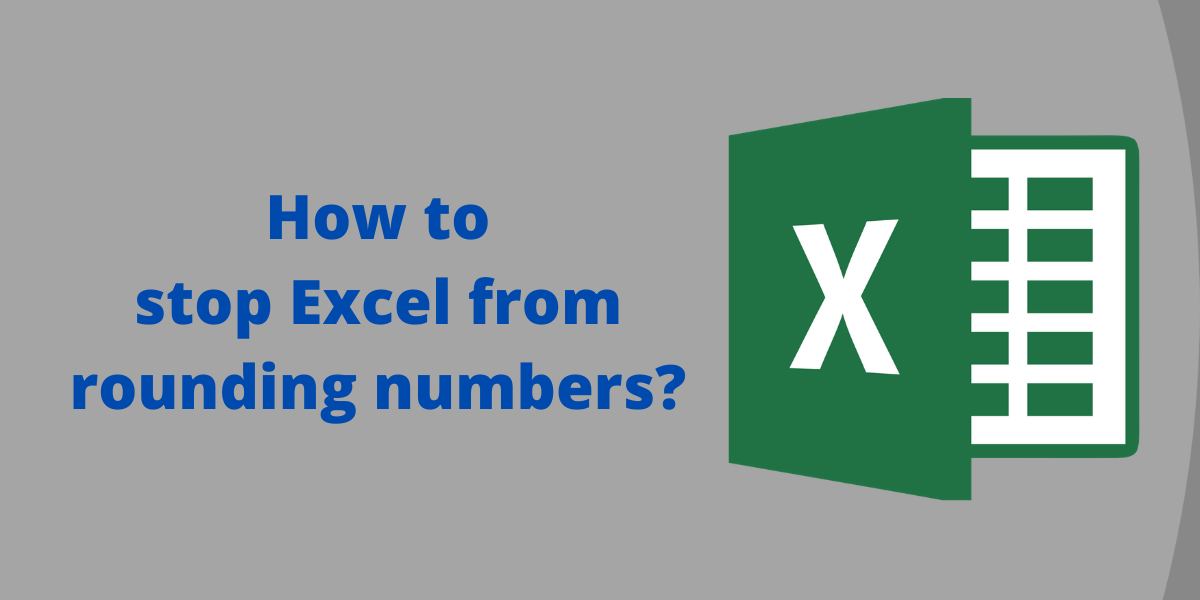# How to stop Excel from rounding numbers?In this article, we’ll learn how to stop Excel from rounding numbers. When you enter a number in a cell, Excel may round it off. It is quite annoying when Excel keeps rounding off numbers. While there may be several reasons behind this but the most common reasons are:

• If the cell width is small, the number is rounded off to fit in the space available.
• The precision for decimal values is set lower than the actual required to display the input number properly.
• The input number is too large with more than 15 digits; Excel rounds off the number to 15 significant digits only.
• The default format for Excel cells is set to General, which is not enough to handle number inputs.

## Stop Excel from rounding large numbers

You may sometimes need to input large numbers with more than 15 digits. In such cases, Excel rounds off the number to 15 significant digits only. The remaining digits are changed to zeroes. This is due to Excel’s limitation of being able to display only 15 digits, by default. Here is an example of how you can stop Excel from rounding off large numbers.

The input number in cell A1 is “123456789098765432”, which contains 18 digits before hitting Enter.

If you hit Enter, cell A1 will display the value to be “123457E+17” while the formula bar displays “123456789098765000”.

To stop Excel from rounding off the digits, do the following:

• Change the format of the cell from General to Text; or
• Put an apostrophe before entering the number in the cell.

## Stop Excel from rounding whole numbers

When you enter a number larger than the Excel cell could accommodate, it is rounded off. Here’s an example:

To avoid this type of rounding,

• Increase the cell width by adjusting the right margin of the cell to display the whole number.

Here is an example of how Excel rounds off decimal numbers: